Letter and Symbol Series

Letter and Symbol Series are a sequential order of letters, numbers or both arranged such a way that each term in the series is obtained according to some specific rules. These rules can be based on mathematical operations, place of letters in alphabetical order etc.

Letter series type of questions usually consists of a series of small letters which follow a certain pattern. However, some letters are missing from the series. These missing letters are then given in a proper sequence as one of the alternatives. The candidate is requitred to choose this alternative as the answer.

Two or three questions from Letter and Symbol Series is there in every exam. In such questions of letter/alphabetic and symbol series, if the question consists of a single letter/ alphabet series, then you have to solve the logic implied in the sequence. Then fill in the missing character with a correct choice. Here , we will discuss alphabetical series tricks.

ALPHABETICAL SERIES TRICKS

A B C D E F G H I J K L M N O P Q R S T U V W X Y Z

1. THE ALPHABET: There are 26 letters in English alphabets.

The first half of alphabet series is from A to M and the half is from N to Z.

A-M – 1-13 (First Alphabetical Half)

N-Z –14-26 (Second Alphabetical Half)

Hence So if you remember these intervals,It would be helpful in solving Letter/Alphabet Series problems.

2. Intervals like: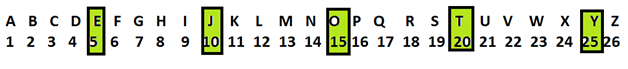I. EJOTY: To make it easier there is a trick called EJOTY (i.e From left to Right) by which you can simply locate the position of letter without much effort.

E      J      O      T         Y

5    10    15     20        25

Suppose we want to find the place of the 20th letter from the left side. And you know 20th letter is T, so now, you only have to find a letter which is two positions ahead of T, which is V.

II. VQLGB: It is similar to EJOTY but this is in reverse order i.e from right to left.

V      Q      L      G         B

5      10   15    20       25

By these simple tricks, you can instantly find the position of any letter either from the left side or the right side without much efforts.This trick will help you to solve basic questions on alphabet series.

3. Most of the questions from Alphabetic series in the exams will be from the right side as we are habitual of counting from the left side i.e A,B,C,D… But before we proceed here are few

MIND IT !

i. From your left implies left to right.

ii. To the right implies from left to right.

iii. To the left implies from right to left.

For example, Let's find the place of a letter from right side which is the 14th from the left side.

TIPS on cracking Reasoning Questions on Letter and Symbol Series

Tip #1: Break the complex letter series into simpler ones and solve them individually

Question: Look at the following series and fill in the blank.

SCD, TEF, UGH, ____, WKL

Solution:

There are two alphabetical series here. The first series is with the first letters only, and consists of the consecutive letters of the alphabet starting from S, i.e., S, T, U, V, W.

The second series involves the remaining two letters and involving 2 consecutive letters of the alphabet starting from C, i.e., CD, EF, GH, IJ, KL. So the missing term will be VIJ.

Question: Look at the following series and fill in the blank.

DEF, DEF2, DE2F2, _____, D2E2F3

Solution:

In this series, the letters remain the same: DEF.

The subscript numbers follow the following series: 111, 112, 122, 222, 223, 233, 333, ...

Thus, the missing term is D2E2F2.

Question: Look at the following series and fill in the blank.

JAK, KBL, LCM, MDN, ____.

Solution:

This series may be broken up into 3 component series. The 1st one is the series J, K, L, M and N. The 2nd one follows the pattern A, B, C, D, E, and the 3rd one: K, L, M, N and O.

Thus the missing term is NEO.

Tip #2: Identify the changes happening in the successive patterns of a symbol series.

Question: Look carefully at the sequence of symbols to find the missing pattern.

Solution: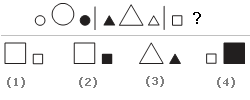In each segment, the shapes are the same, but the one in the middle is larger than the two on either side. Also, one of the figures is shaded and that this shading alternates between 1st right and then left. Thus in the 3rd segment, there will be two small squares with a big square between them and the right small square will be blackened. Thus, the correct option is (2).

Question: Look carefully at the sequence of symbols to find the missing pattern.

Solution: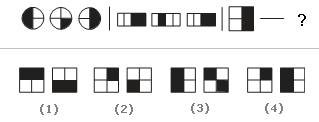In each of the segments, the figures alternate between one-half and one-fourth shaded on the opposite sides. Thus, to complete the last segment, there should be one square that is one-fourth shaded and another that is half-shaded on the left side. Thus, the correct option is (4).

Tip #3: Eliminate the incorrect choices

Question: Look carefully at the sequence of symbols to find the missing pattern.

Solution: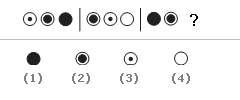Since no 2 figures in the same segment are the same, Options (1) and (2) are eliminated. Now, the shading inside the circles gradually increases or decreases. Thus, the correct option is (3).

Question: Look at the following series and fill in the blank. JAK, KBL, LCM, MDN, _____

A. OEP    B. NEO    C. MEN    D.PFQ

Solution:

The first letter of each term follows the series J, K, L, M and N. This eliminates choices A, C, and D. Thus, the correct answer is B.

Question: Look carefully at the sequence of symbols to find the missing pattern.

Solution: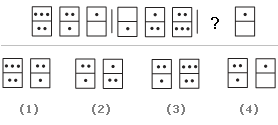In all these segments, the count of dots in the bottom box of the previous set equals the count of dots in the top box of the current set. This eliminates options (2), (3) and (4). Thus, the correct option is (1).